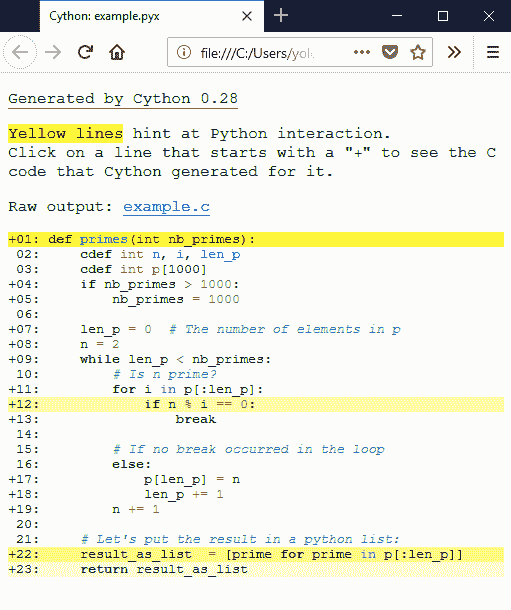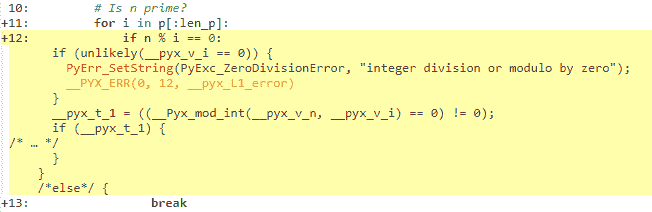# 基础教程

## Cython基础

Cython 的本质可归纳为：Cython 是具有 C 数据类型的 Python。

Cython 是 Python：几乎任何 Python 代码都是有效的 Cython 代码。 （有一些 限制 ，目前还只是“几乎”）Cython 编译器将其转换为 C 代码，它对 Python / C API 进行等效调用。（译者注：目标是完全兼容）

## Cython Hello World

print("Hello World")



from distutils.core import setup
from Cython.Build import cythonize

setup(
ext_modules = cythonize("helloworld.pyx")
)



$python setup.py build_ext --inplace  在unix中，这将在您的本地目录中创建 helloworld.so （Windows 中为 helloworld.pyd ）。现在使用这个文件：启动 python 解释器并简单地导入它就好像它是一个普通的 python 模块： >>> import helloworld Hello World  恭喜！您现在知道如何构建 Cython 扩展。但到目前为止，这个例子并没有真正让人感觉为什么会想要使用 Cython，所以让我们创建一个更现实的例子。 ### pyximport：面向开发人员的 Cython 编译 如果您的模块不需要任何额外的 C 库或特殊的构建设置，那么您可以使用最初由 Paul Prescod 开发的 pyximport 模块在导入时直接加载.pyx 文件，而无需在每次你修改完代码之后运行setup.py。它随 Cython 一起发布和安装，可以像这样使用： >>> import pyximport; pyximport.install() >>> import helloworld Hello World  Pyximport 模块还具有对普通 Python 模块的实验性编译支持。这允许您在 Py​​thon 导入的每个.pyx 和.py 模块上自动运行 Cython，包括标准库和已安装的软件包。 Cython 仍然无法编译很多 Python 模块，在这种情况下，导入机制将回退到加载 Python 源模块。 .py 导入机制安装如下： >>> pyximport.install(pyimport=True)  请注意，建议不要让 Pyximport在最终用户端构建代码，因为它会挂钩到他们的导入系统。满足最终用户的最佳方式是以wheel包的格式提供预先构建的二进制包。 ## 斐波那契函数的乐趣 官方 Python 教程中有一个简单的 fibonacci 函数，定义为： from __future__ import print_function def fib(n): """Print the Fibonacci series up to n.""" a, b = 0, 1 while b < n: print(b, end=' ') a, b = b, a + b print()  现在按照 Hello World 示例的步骤，我们首先将文件重命名为 pyx 扩展名(假设是 fib.pyx )，然后我们创建 setup.py 文件。使用为 Hello World 示例创建的文件，您需要更改的是 Cython 文件名的名称，以及生成的模块名称，我们这样做： from distutils.core import setup from Cython.Build import cythonize setup( ext_modules=cythonize("fib.pyx"), )  使用与 helloworld.pyx 相同的命令构建扩展模块： $ python setup.py build_ext --inplace



>>> import fib
>>> fib.fib(2000)
1 1 2 3 5 8 13 21 34 55 89 144 233 377 610 987 1597



## 素数

primes.pyx

 def primes(int nb_primes):
cdef int n, i, len_p
cdef int p
if nb_primes &gt; 1000:
nb_primes = 1000

len_p = 0  # The current number of elements in p.
n = 2
while len_p &lt; nb_primes:
# Is n prime?
for i in p[:len_p]:
if n % i == 0:
break

# If no break occurred in the loop, we have a prime.
else:
p[len_p] = n
len_p += 1
n += 1

# Let's return the result in a python list:
result_as_list  = [prime for prime in p[:len_p]]
return result_as_list


cdef int n, i, len_p
cdef int p



if nb_primes > 1000:
nb_primes = 1000



len_p = 0  # The number of elements in p
n = 2
while len_p < nb_primes:



# Is n prime?
for i in p[:len_p]:
if n % i == 0:
break



for i in p[:len_p]:



# If no break occurred in the loop
else:
p[len_p] = n
len_p += 1
n += 1



# Let's put the result in a python list:
result_as_list  = [prime for prime in p[:len_p]]
return result_as_list



>>> import primes
>>> primes.primes(10)
[2, 3, 5, 7, 11, 13, 17, 19, 23, 29]



Cython 有一种方法可视化与 Python 对象和 Python 的 C-API 进行交互的位置。为此，将annotate=True参数传递给cythonize()。它生成一个 HTML 文件。让我们来看看：def primes_python(nb_primes):
p = []
n = 2
while len(p) < nb_primes:
# Is n prime?
for i in p:
if n % i == 0:
break

# If no break occurred in the loop
else:
p.append(n)
n += 1
return p



from distutils.core import setup
from Cython.Build import cythonize

setup(
ext_modules=cythonize(['example.pyx',        # Cython code file with primes() function
'example_py_cy.py'],  # Python code file with primes_python_compiled() function
annotate=True),        # enables generation of the html annotation file
)



>>> primes_python(1000) == primes(1000)
True
>>> primes_python_compiled(1000) == primes(1000)
True



python -m timeit -s 'from example_py import primes_python' 'primes_python(1000)'
10 loops, best of 3: 23 msec per loop

python -m timeit -s 'from example_py_cy import primes_python_compiled' 'primes_python_compiled(1000)'
100 loops, best of 3: 11.9 msec per loop

python -m timeit -s 'from example import primes' 'primes(1000)'
1000 loops, best of 3: 1.65 msec per loop



primes_python的 cythonize 版本比 Python 版本快 2 倍，而不需要更改单行代码。 Cython 版本比 Python 版本快 13 倍！有什么可以解释这个？

• 在这个程序中，每行都进行很少的计算。因此 python 解释器的开销非常重要。如果你要在每一行做很多计算，那将会非常不同。以 NumPy 为例。
• 数据局部性。使用 C 时，使用 Python 时可能会有更多的内容适合 CPU 缓存。因为 python 中的所有内容都是一个对象，并且每个对象都是作为字典实现的，所以这不是很容易缓存的。

## 使用 C ++ 语言

# distutils: language=c++

from libcpp.vector cimport vector

def primes(unsigned int nb_primes):
cdef int n, i
cdef vector[int] p
p.reserve(nb_primes)  # allocate memory for 'nb_primes' elements.

n = 2
while p.size() &lt; nb_primes:  # size() for vectors is similar to len()
for i in p:
if n % i == 0:
break
else:
p.push_back(n)  # push_back is similar to append()
n += 1

# Vectors are automatically converted to Python
# lists when converted to Python objects.
return p